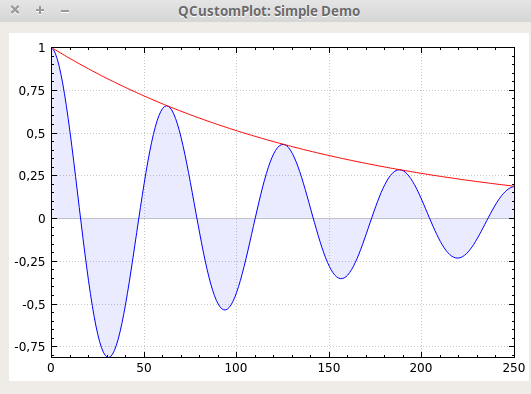# Do I need to use threading?

• I'm currently using qcustomplot (https://www.qcustomplot.com/index.php/demos/simpledemo) to plot some curves on a window, like the following,but what I'm trying to do is to change the values of the vector representing the y axis, plot them, and doing that each 1 second.
Suppose for example that we have Qvector<int> x, as increasing integers from 0 to 250, and QVector<int> y as x², 0², 1², 2² ....etc, then each second I want to add noise to each y[i] and plot it. Therefore we wil have a noisy curve changing each second.

In order to do that each second, I need to use the sleep() function from <unitstd.h>, but this function prevents the window from opening. Therefore I thought it's because the program is stuck 99.99 % of the time on the sleep function, maybe I need another thread that sleeps and loads the data, and another that displays the window?

• you use qtimer(not sleep()) to do it. Then no thread is needed.

• you use qtimer(not sleep()) to do it. Then no thread is needed.